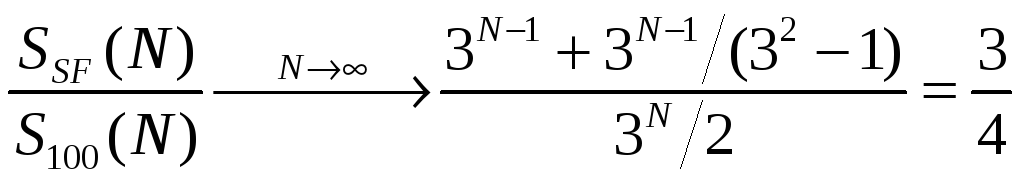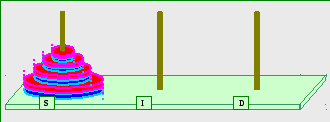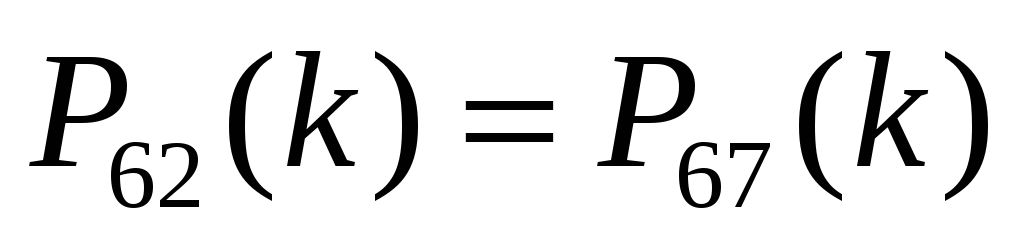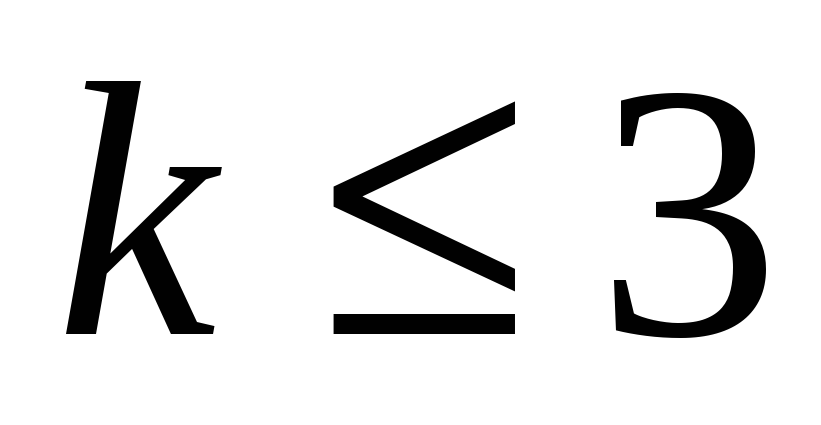# The Magnetic Tower of Hanoi

 Page 10/20 Date 23.04.2018 Size 2.78 Mb. #45758

Table 8: Number of disk-moves for the "SemiFree" Algorithm of the Magnetic Tower of Hanoi puzzle (Figure 10). N is the total number of disks to be moved from Post S to Post D, and k is the disk number in the ordered stack, counting from bottom to top. Equation 15 spells out the PSF(k) calculating expression and Equation 16 spells the expression for calculating the sum - SSF(N). Compare the numbers in this "SemiFree" table to the smaller corresponding numbers in the "67" table (Table 6) but Compare the numbers in this "SemiFree" table to the much larger corresponding numbers in the "100" table (Table 4), to realize the "SemiFree" savings.
With Equation 16 in place, we can easily find the limit of the "duration-ratio" of "SF" vs. "100" as follows:(17)
The curve for the duration-ratio of "SF" vs. "100" (along with similar curves for "67" vs. "100" and "62" vs. "100") is shown in Figure 12 below.

Standing on top of the SemiFree "hill", we can already see the "62" summit. Let's then, following a short rest, climb the last mile.

1. The "62" Algorithm

With the SemiFree Algorithm in place, along with the "100" Algorithm and the "67" Algorithm, we now return to the original MToH and swiftly solve the puzzle.Figure 11: The "regular" or "Free" MToH puzzle. The diagram shown in the figure is just a copy of the diagram shown in Figure 2, placed here for clarity and for reader's convenience.
Figure 11 is just a copy of Figure 2, placed here for reader's convenience. Let' also repeat the game's objective - we want to efficiently relocate (i.e. relocate by a small number of moves) the N disks placed originally over the Source-Post onto the Destination-Post, subject to the Size Rule as well as to the Magnet Rule. To accomplish this mission (solve the puzzle efficiently), we present the "62" Algorithm.

Described in very general terms, the "62" Algorithm is made up of three steps –

• Move N-1 disks down onto Post I, colored Blue at the end of the sequence, using the "67" Algorithm

• Move two more disks to Post D while leaving N-3 disks on Post I, colored Red at the end of the sequence, using essentially the SemiFree Algorithm

• Move up the remaining N-3 disks (from Post I to Post D), using again the "67" Algorithm

An accurate, more detailed, description of the "62" Algorithm is given in Table 8.

 Step # Disks From To # of moves Comments 1 2 to N S (Red) I (Blue) S67(N-1) Going "down" 2 1 S (Red) D (Blue) 1 3 3 to N I (Blue) D (Blue) 2*S100(N-2) Start folding up here 4 2 I (Blue) S (Red) 1 5 4 to N D (Blue) S (Red) SSF(N-3) SemiFree Algorithm 6 3 D (Blue) I (Red) 1 Post I changed color 7 4 to N S (RED) I (Red) 2*S100(N-3) N-2 disks on Post I 8 2 S(RED) D(Blue) 1 9 3 to N I (Red) D (Blue) S67(N-2) Efficient up-folding

Table 8: The "62" Algorithm for N ≥ 3. For N < 3, the"62" Algorithm coincides with the "67" Algorithm (see Table 5). The "62" Algorithm involves all three algorithms already analyzed – "100", "67", and "SemiFree".
Note that two "67" Algorithms are used in the "62" solution sequence. The one in step 1 is actually "67-Down" Algorithm. The one in step 9 is actually "67-Up" Algorithm. The "67-Up" Algorithm is a "time-reversed" "67-Down" Algorithm (and vice-versa – see Appendix 1). Necessarily, the move-counting equations (Equations 10 and 12) apply equally well to both algorithm variations.
We want now to develop expressions for the number of puzzle-solving moves, for the "62" Algorithm. Looking at Table 8 we see only "recognized" algorithms ("100", "67", and "SemiFree"). Expression for the number of moves of the k-th disk for each of the three participating algorithms was already presented above. Similarly for the total number of moves. So now, for the "62" Algorithm, we simply sum the previously developed expressions -;